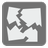Weak Point
Elementary
English FR RU UK

While traveling, the spaceship endures quite a lot of stress. As a result, an important part of the maintenance is to check the outer hull. Stephan uses a digital durabilitimeter for this task. The device scans a portion of the spaceships hull and gives a durability map that is divided by small square fragments with measurements. Sometimes Stephan does not have much time and he can patch only couple points, so we need an algorithm to find the weak points.

The durability map is represented as a matrix with digits. Each number is the durability measurement for the cell. To find the weakest point we should find the weakest row and column. The weakest point is placed in the intersection of these rows and columns. Row (column) durability is a sum of cell durability in that row (column). You should find coordinates of the weakest point (row and column). The first row (column) is 0th row (column). If a section has several equal weak points, then choose the closest point to the top edge and if there are multiple points closest to the top edge, then choose from those points the point that is closest to the left edge.Input: A durability map as a list of lists with integers.

Output: The coordinates of the weak point as a list (tuple) of integers.

Example:

```weak_point([[7, 2, 7, 2, 8],
[2, 9, 4, 1, 7],
[3, 8, 6, 2, 4],
[2, 5, 2, 9, 1],
[6, 6, 5, 4, 5]]) == [3, 3]
weak_point([[7, 2, 4, 2, 8],
[2, 8, 1, 1, 7],
[3, 8, 6, 2, 4],
[2, 5, 2, 9, 1],
[6, 6, 5, 4, 5]]) == [1, 2]
```

How it is used: Matrices (2D array) are an often used data structure and it will be helpful to sharpen your skills with them.

Precondition:
0 < len(matrix) ≤ 10
all(len(row) == len(matrix) for row in matrix)
all(all(0 < x < 10 for x in row) for row in matrix)